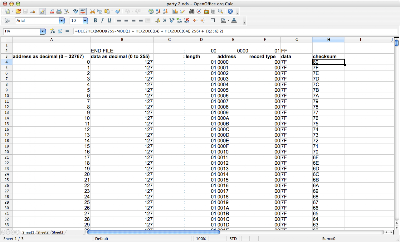## Wednesday, November 12, 2008

### Formatting Intel HEX Files from a Spreadsheet

I have a need to convert data from a spreadsheet into an Intel HEX file. Although it may seem a little impractical, I decided to use one record entry per data byte, because this made it a little easier (and also, I don't need the hex file itself to be small and efficient - I just need it to work). It has space for 32KB worth of actual data in the hex file.

Here you can see a snapshot of my spreadsheet (from OpenOffice):Column A is the address as a decimal (from 0 to 32767).

Column B contains my data as a decimal (with the range 0 to 255).

Column C is the colon which marks the beginning of each record entry in a hex file. This is where the formatting for the hex file begins.

Column D is the length field for each entry, which in this case is always set to \$01.

Column E is the address as a four digit hexadecimal number (\$0000 to \$ffff). This is referenced from Column A using DEC2HEX(An; 4), where n is the current row number.

Column F is the record type field for each entry, which in this case is always set to \$00 (this indicates a data record).

Column G is the data field as a two digital hexadecimal number (\$00 to \$ff). This is referenced from Column B using DEC2HEX(Bn; 2), where n is the current row number.

Column H is the checksum as a two digital hexadecimal number. This is calculated as:
=DEC2HEX(MOD((255-MOD((1 + HEX2DEC(En) + HEX2DEC(Gn));256) + 1);256);2), where n is the current row number.

Then it is just a matter of copying and pasting the cell range C4:H32771 into a text file, adding the END FILE entry (cell range C2:G2 or simply :00000001FF) and replacing all spaces with nothing.